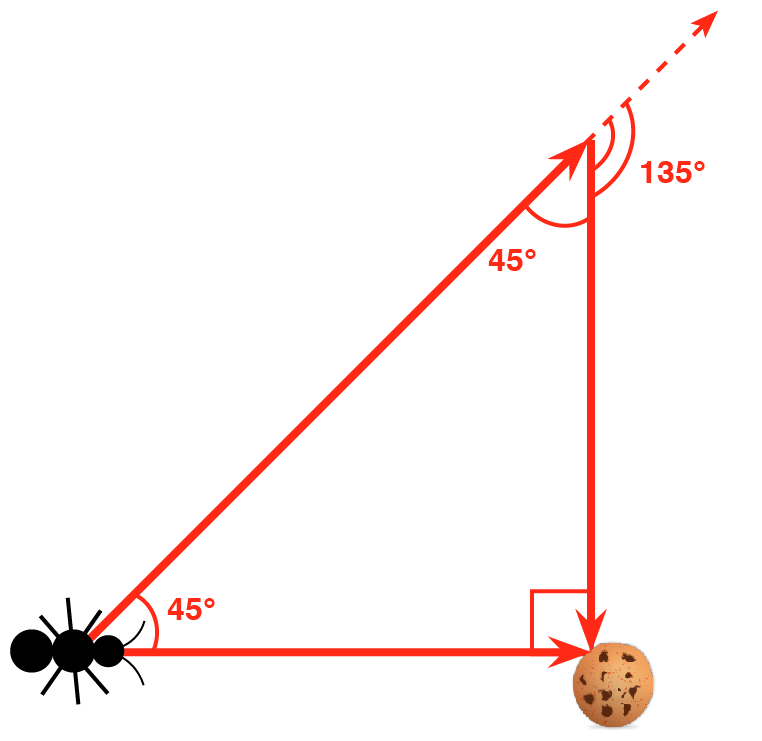# Word Problem Wednesday: Ants!

By Damaris | Added Dec 7, 2016For this installment of Word Problem Wednesday, we're challenging you to use your high school geometry knowledge to solve a creepy crawly word problem! Give it a try and check back tomorrow for the answer.

Believe it or not, ants use math to get food efficiently. Instead of following the shortest path to food, ants take the path that will take the least time. Please refer to the diagram above. The shortest route from the ant to the cookie is two inches. Because of the terrain, the ant can get to the cookie faster if it turns 45° to the left and then 135° to the right. Exactly how much greater is the distance of the path that takes less time?

Update: Here's the solution!

To find the length of each side of the triangle, we can use the measures of the interior angles. This is a 45°—45°—90° triangle. This is an isosceles right triangle; two of its angles are equal and two of its sides are equal.
To find the length of the hypotenuse, we can use the Pythagorean theorem: 2² + 2² = c², 8 = c², c = 2sqrt(2). We can also use the 45°—45°—90° triangle rule—the hypotenuse is equal to a leg multiplied by the square root of 2. The longer route is 2sqrt(2) + 2 inches and the shorter route is 2 inches. The difference is therefore 2sqrt(2) inches.